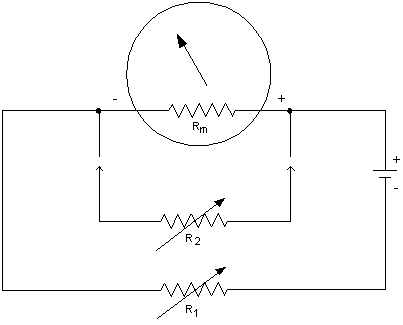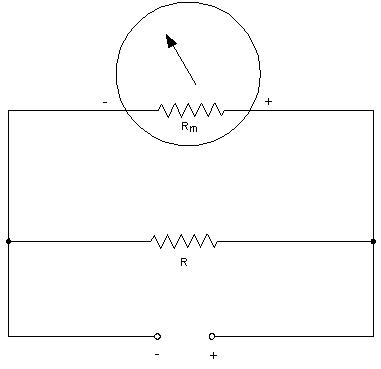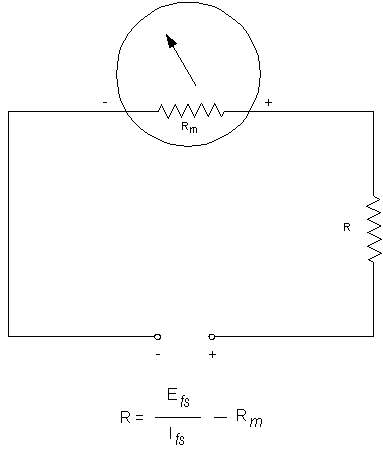Kits > General Info > DC Meter Formulas

Nostalgic Kits Central

# DC Meter Formulas

Meter Resistance  Current Shunt  Voltage Multiplier  Ohms per Volt

There may be times when a defective panel meter needs repair or replacement. As original meters can be difficult to find, a replacement may have to be made from non-original stock. To help with this process, the following information is provided.

### Meter Resistance

To determine the internal resistance of a meter, perform the following steps:1. Connect the meter in series with a DC power source (battery) and a variable resistance R1.
2. Starting from highest resistance, vary R1 until you attain a full scale reading.
3. Connect a second variable resistor R2 across the meter. Vary R2 untilyou attain a half scale reading.
4. After disconnecting R2 from the circuit, measure its resistance. It will be equal to the meter resistance Rm.

Note: Insure that R1 is of high enough a value to prevent allowing too much current to flow through the meter. Use the following formula to estimate a safe value for R1:### Current Shunt

Use the following formula to calculate the shunt resistance for a current meter:R = Rm/N - 1
 Where: R = shunt value in ohms N = new full scale reading divided by original full scale reading Rm = meter resistance in ohms

### Voltage Multiplier

Use the following formula to calculate the series resistance for a volt meter:Where: R = series multiplier value in ohms Efs = full scale reading in volts Ifs = full scale current of meter in amps Rm = meter resistance in ohms

### Ohms per VoltWhere: Ifs = full scale current in amps

Last update: 16 Aug. 2003